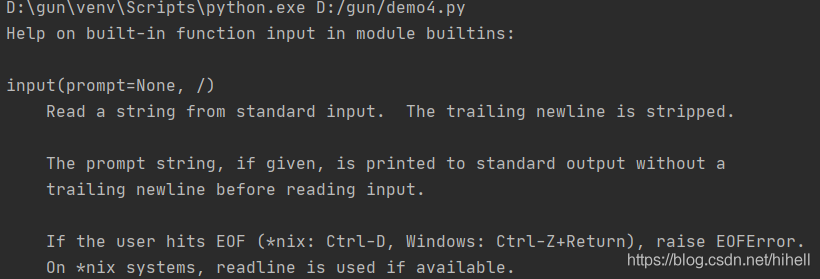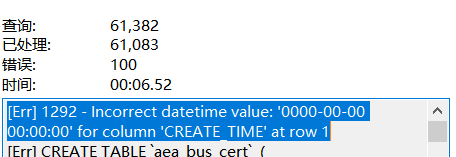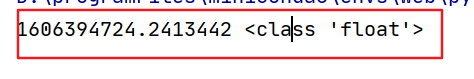2. 无门槛学会数据类型与输入、输出函数，滚雪球学 Python

# 二、Python 基本数据类型与输入、输出函数

## 2.1 基本数据类型

1. 数值数据类型，常见的是整数和浮点数
2. 布尔值数据类型，就是常说的真和假
3. 字符串数据类型

``````x = 10
print(type(x))``````

``<class 'int'>``

``````x = 10.1
print(type(x))
``````

``<class 'float'>``

### 2.1.1 数值数据类型

``````x = 10.1
y = 10
z = x + y
print(type(z))``````

### 2.1.2 整数的不同形式表示

``````x = 10
print(bin(x))``````

``0b1010``

### 2.1.3 数据类型强制转换

``````x = 10.5
print(int(x))``````

int 其实也是 Python 的一个内置函数，它会尝试将任何数据类型的变量转换成整数，注意是任何数据类型的变量。

### 2.1.4 扩展几个数值数据类型常用的函数

• abs() 计算绝对值
• pow() 次方运算
• round() 四舍五入
• max() 取最大值
• min() 去最小值

``````# abs() 计算绝对值
a = -1
print(abs(a))
# pow() 次方运算
x = 2
c = 3
print(pow(x,c))
# round() 四舍五入

d = 34.6
print(round(d))
# max()  取最大值
print(max(1,2,3))
# min() 去最小值
print(min(9,10,6))
``````

### 2.1.5 布尔值数据类型

``````x = True
print(int(x))
print(int(False))``````

### 2.1.6 字符串数据类型

``````my_name = "梦想橡皮擦"
print(my_name)

my_name = '梦想橡皮擦'
print(my_name)

my_name = '梦想"橡皮擦'
print(my_name)
print(type(my_name))``````

``````a = "my"
b = "name"

c = a+b
print(c)``````

``````a = 123
b = "name"
# str(a) 将整数变量a转换成字符串
c = str(a) + b
print(c)
``````

``````my_str = """

"""
print(my_str)``````

``````a = 'I\'m a girl '
print(a)
``````

``````a = r"I\nm a girl"
print(a)``````

``I\nm a girl``

``print("#"*100)``

## 2.2 输入与输出

``help(print)``

``print(value, ..., sep=' ', end='\n', file=sys.stdout, flush=False)``

• value 表示要输出的数据，可以多个，用逗号 `,` 分隔
• sep 输出多个数据时，分隔符号，默认是空格
• end 输出一行末尾输出的符号
• file 输出位置，默认输出控制台，就是黑窗口中，可以设置到具体文件
• flush 是否清除数据流缓冲区，默认为 False（该内容先不做涉及）

``````# 输出多个数据
print("hello","dream")

# 多个数据输出的分隔符
print("i","love",sep="#")``````

### 2.2.1 格式化 print 输出

• `%d` 整数输出
• `%f` 浮点数输出
• `%x` 十六进制输出
• `%o` 八进制
• `%s` 字符串输出，其实会这一个就行了

``````name = "橡皮擦"
age = 18
score = 100

# 格式化一个变量输出
print("我是 %s" % name)
# 格式化多个变量输出
print("我是 %s 今年 %d岁了，我考试得了%d分" % (name,age,score))
``````

``````name = "橡皮擦"
age = 18
score = 100

print("我是 %s" % name)
print("我是 %s 今年 %d岁了，我考试得了%d分" % (name,"nnn",score))
``````

``````name = "橡皮擦"
age = 18
score = 100

print("我是 %s" % name)
print("我是 %s 今年 %s岁了，我考试得了%d分" % (name,18,score))``````

### 2.2.2 format 函数

``print("我是{}，今年{}，考试得了{}".format(name,age,score))``

### 2.2.3 input 数据输入

input 执行的和 print 恰好想法，通过 help 查看一下：参数比较简单只有一个输入提示语。测试代码：

``````name = input("请输入你的姓名：")
print("输入的姓名为{}".format(name))``````

`input` 比较简单，本文先介绍这些。

## 2.3 这篇博客的总结

`好好学下去 每天都有新打击`O(∩_∩)O 哈哈~blmius
2年前
MySQL:[Err] 1292 - Incorrect datetime value: ‘0000-00-00 00:00:00‘ for column ‘CREATE_TIME‘ at row 1Jacquelyn38
2年前
2020年前端实用代码段，为你的工作保驾护航helloworld_34035044
1年前

​在我们用皕杰报表工具设计填报报表时，如何在新增行里自动增加id呢？能新增整数排序id吗？目前可以在新增行里自动增加id，但只能用uuid函数增加UUID编码，不能新增整数排序id。uuid函数说明：获取一个UUID，可以在填报表中用来创建数据ID语法：uuid()或uuid(sep)参数说明：sep布尔值，生成的uuid中是否包含分隔符''，缺省为Karen110
2年前
​一篇文章总结一下Python库中关于时间的常见操作Stella981
2年前
Python3：sqlalchemy对mysql数据库操作，非sql语句
Python3：sqlalchemy对mysql数据库操作，非sql语句python3authorlizmdatetime2018020110:00:00coding:utf8'''Stella981
2年前
Python之time模块的时间戳、时间字符串格式化与转换
Python处理时间和时间戳的内置模块就有time，和datetime两个，本文先说time模块。关于时间戳的几个概念时间戳，根据1970年1月1日00:00:00开始按秒计算的偏移量。时间元组（struct_time），包含9个元素。 time.struct_time(tm_yWesley13
2年前
mysql设置时区
mysql设置时区mysql\_query("SETtime\_zone'8:00'")ordie('时区设置失败，请联系管理员！');中国在东8区所以加8方法二：selectcount(user\_id)asdevice,CONVERT\_TZ(FROM\_UNIXTIME(reg\_time),'08:00','0Wesley13
2年前
00：Java简单了解Stella981
2年前Wesley13
2年前
MySQL部分从库上面因为大量的临时表tmp_table造成慢查询Lv1

15

1

3